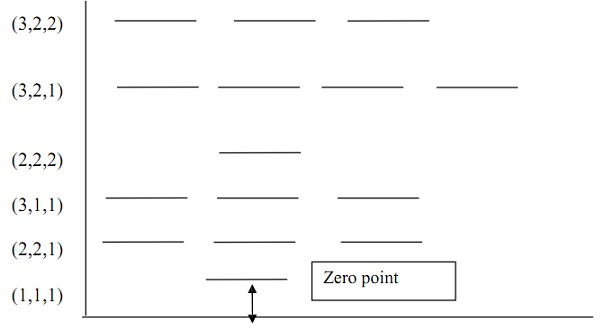#### Particle in Three-Dimensional Box, Chemistry tutorial

Introduction

Suppose we consider the similar particle as in chapter but this time it is constrained to shift in a rectangular box of dimensions a, b and c in length. Within the box (i.e. between x= 0 and a; y = 0 and b and z = 0 and c), the potential energy is zero. At the walls and everywhere outside the box, the potential is.

Definition of Particle in a Three-Dimensional Box

The Schrodinger wave equation for 3 dimensional (3D) box is

δ2φ/δx2 + δ2φ/δy2 + δ2φ/δz2 + 8 π2m/h2(E-V) φ =0

where   and V are  (x,y,z).

since V = 0 inside the box, then the equation becomes

δ2φ/δx2 + δ2φ/δy2 + δ2φ/δz2 + 8 π2m/h2Eφ=0

Equation may be solved by writing the wave function as the product of 3 functions, each depending on one coordinate.

φ(x, y, z) = X(x)Y(y)Z(z)

differentiating equation gives

δφ/δx = Y (y) Z (z) δx/δx

δ2φ/δx2= Y (y) Z(z)  δ2x/δx2

and by a similar reasoning

δ2φ/δy2 = X (x)Z(z) δ2y/δy2

δ2φ/δz2 = X(x)Y(y) δ2z/δz2

Y(y)Z(z)δ2x/δx2 + X(x)Z(z) ) δ2y/δy2 + X(x)Y(y) δ2z/δz2 + 8 π2m/h2 EX(x)Y(y)Z(z) = 0 dividing all through by Y(y)X(x)Z(z) one obtains

-h2/8 π2 m* 1/X(x) δ2x/δx2 +1/Y(y) * δ2y/δy2   + 1/Z(z) δ2z/δz2 = E

We can write the energy as the sum of three contributions associated with the coordinates.

E = Ex + Ey + Ez

using equation, we can divide the expression attained into 3 equations.

-h2/8 π2 m *1/x δ2x/δx2 = Ex

-h2/8 π2 m *1/y δ2y/δy2 = Ey

-h2/8 π2 m *1/z δ2z/δz2 = Ez

Each of the last three equations is similar to the expression for the particle in  a  one-dimensional  box  discussed  in  chapter.  Hence their solutions are respectively:

X = [√(2/a)]sin nπx/a

En,x =  n2xh2/8ma2

Y =  [√(2/b)]sin nπy/b

En,y  = n2y h2/ 8mb2

X = [√(2/c)] sin nπx/c

En,z =  n2z h2/ 8mc2

Where a, b, c are length in x, y, z directions, correspondingly. Also, nx, ny, nz are the quantum numbers correspondingly.

Since φ (x, y, z) = X(x)Y(y)Z(z) and E = Ex  + Ey + Ez, then

ψ (x,y,z) = √a/v sin(nπx/a) sin(nπy/b) sin(nπz/c)

where V is the volume of the box.

Ex,y,z  =  h2/δm * n2x/a2 + n2y/b2 [+ n2z/c2]

Whenever the three dimensional box has geometrical symmetry, more interesting results are often obtained. For example, in a cubic box, a = b =c, thus equation becomes

E = h2/ φm(n2x + n2y + n2z)

Suppose nx =3, ny = nz =2, then

Ψ(x,y,z) = [/(8/v)] sin3πx/a* sin2πy/a*sin2πz/a]

E =  h2/8ma2(32 + 22+ 22) =  17h2/8ma2

Assuming we have another set of values nx =2, ny =3, nz  =2, then

Ψ(x,y,z) = [/(8/v )]sin 2πx/a sin3πy/a  sin2πz/a

E =   h2/8ma2 (22 + 32+ 22) = 17h2/8ma2

Suppose nx =2, ny =2, nz =3, then

Ψ(x,y,z) = [/( 8/v)]sin2πx/a sin2πy/a sin3πz/a

E = h2/8ma2(22 + 22+ 32) = 17h2/8ma2

Even though such states are different, the values of the energies are the same. The three states are said to be degenerate since they have equal energy.

For a situation where nx = ny = nz = 1, it corresponds to only one state of the system. The same is true of nx = ny = nz = 2, but situations such as nx ≠ ny = nz three degenerate states are obtained as shown in the Figure below.Fig: Quantized Energy Levels of a Particle in a Cubic Box

Suppose we wish to calculate the transition energy between the levels E2,2.2  and E3,2,1  then the energy difference is calculated as

ΔE = hν = 14h2/8ma2 - 12h2/8ma2 = 2h2/8ma2 = h2/4ma2

Given suitable data, it should be possible for us to calculate the frequency (ν) of the transition between the two states.

Zero point energy

According the old quantum theory, the energy level of a harmonic oscillator is E = hv.

The lowest energy level via n = 0 would have zero energy. Depend on the wave treatment of the system, the energy level corresponds to the state with quantum numbers nx = ny =  nz =1. The dissimilarity between such 2 values is termed the zero point energy.

Tutorsglobe: A way to secure high grade in your curriculum (Online Tutoring)